# buck converter simulation, some insights and curiosities

#### ag-123

Joined Apr 28, 2017
273
I've updated my diy "buck converter simulator" that basically integrates the ODE of the few components, this time showing the Vin, inductor currents and dVout/dt - a function of the capacitor current.
https://github.com/ag88/jbuckconv/releases/tag/v0.2.0
https://github.com/ag88/jbuckconv

The results are somewhat interesting, if a little surprising.
it turns out that the load needs to draw quite some currents for that inductor to look like "continuous conduction mode".
And a quite sizable inductor is needed as if it is too small, the capacitor seemed to dominate.
Note that a diode is not simulated in this study, it is simply treated as 2 phases
On phase, Vin charges up the L, C, R buck converter.
Off phase, Vin is 0, it looks like L, C, R in parallel, Vout is now the buck output voltage

in this simulation, the inductor is 1 mH, capacitor 10 uF, R 10 ohms (load) and Vin 10 V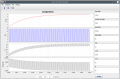If, however, I used a tiny load R = 1000, L 100 uH, C 100 uF, something a little "surprising" is observed. The inductor currents is negative !
In a real buck converter, I'd think this is unlikely, as there is a diode there ! :/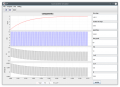It seemed in this case, there is very little energies stored in the inductor as the currents are small R = 1000 ohms and the RC part dominates the circuit behaviour. i.e. it is pretty much charging the capacitor and discharges across R (load), and across the inductor !
er erm well, it is 'wrong'. well, ok. I'm still struggling and i'd try to model the diode#### ag-123

Joined Apr 28, 2017
273
A thing is, the graphs still looked quite different from the deemed currents in steady state analysis
not sure where I goofed, or if what is observed in my "simulator" is after all a "real" behavior?

I'm thinking it could be 'initial conditions', it started with all zeros. That seemed to work ok, but between the high and low Vin levels, I actually did not 'tweak' anything, it is one same equation and I simply let the Runge Kutta integrator do the computation. The ODE equation involved is simply:
$V_{in} = L C \frac{d^2 V_o}{dt^2} + \frac{L}{R} \frac{d V_o}{dt} + V_o \\ where V_o = V_r = V_c$
diode is not there yet. Vin is the driving PWM voltage.

#### ci139

Joined Jul 11, 2016
1,873
Nice attempt . . . i thought to make (mostly) the Ge transistors simulator based only on the real-life measured response of them - but there has been not enough free time for

#### ag-123

Joined Apr 28, 2017
273
It turns out that the little buck converter that looks so "simple", just RLC and pulsed PWM square waves, is literally more complicated than it looks. That "inductor currents" feel real if I used a small R and hence increase the load currents.
And it do behave as what you would for a "real" buck converter, i.e. if I vary the duty cycle, Vout varies according to the duty cycle.
As some parameters, and results look "real" for now, I'm still trying to figure out if I missed anything after all.
I'm thinking if i could "fix" that by modelling the diode, as it need to react to that "negative" diode current which is an "impossibility".
i.e. the diode should look like a very high resistance when Vin is low so that the negative capacitor current should basically just supply the load R.

The app is in the links above in the 1st post in the thread, hence one could download it and try it out. The source codes is in the github repository, so one could checkout the sources and perhaps build it from source and play with it.

Last edited:

#### ag-123

Joined Apr 28, 2017
273
I made an update version 0.2.1. I added diode simulation in the calcs
https://github.com/ag88/jbuckconv
there is no release for this version, considered as alpha codes, to try this out, you would need to clone the repository or download the sources and follow the instructions in the README.md to built it.
The ODE model, with/without diode is accessible on the "compute" menu.

What is more interesting this time is that at 50% duty cycle, at light loads the output voltage Vout is 6v for a 10v Vin.
The 'expected' voltage is 5v, see a little below.
This is likely the case as the negative currents that is flowing through the "inductor", is now "forced" to only flow through the load in the off cycle. Hence, the capacitor only discharges through the load off cycle.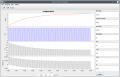if the load is increased R=10 ohm, what is observed is that the Vout now approaches the 'expected' 5v.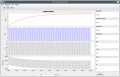and it takes pretty high load (high current drawn) R = 1 ohm, to make it look like "continuous conduction mode"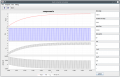the onset of "continuous conduction mode" for smaller loads it seemed can apparently be made by using larger inductors. Nevertheless, the loads need to be quite a bit before the inductor seem to play a part in the "continuous conduction mode", light loads R=1000 and R=100 show very little response or require huge inductors.

I'm not sure if they are after all "real"

Last edited:

#### ag-123

Joined Apr 28, 2017
273

While debugging some issues, I noticed that there seemed to be some errors / inaccuracies with the integration algorithm.
It didn't seem to handle the dV/dt component correctly. Hence, I replaced the integration algorithm with
runge-kutta-nystrom method, that more accurately handles 2nd order ODE.
https://willbeason.com/2021/06/24/introduction-to-runge-kutta-nystrom-methods/

I got a surprise: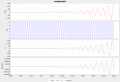I paused to think for a while if this is plausible for a 'buck converter', at least that ODE.
It turns out that this is likely possible, the inductor / capacitor setup can oscillate as like an LC tank circuit at resonance, and it probably reflect the natural frequency of the LC tank circuit.
The Vout doesn't seem realistic, but I'm not too sure if that may after all be plausible.
As the ODE is idealized, real world circuits would likely have attenuations that prevent such large oscillations.

This update is not in the public github version.
https://github.com/ag88/jbuckconv
The github version, still use algorithms available from apache commons math.
https://commons.apache.org/proper/commons-math/
that produce graphs earlier. The issue is the commons-math algorithm currently has some limits in terms of passing the dV/dt component which seemed to be reset during calcs.

#### ag-123

Joined Apr 28, 2017
273
another attempt with the RKN solver

This looks more like previous results, a visible difference is the runge-kutta-nystrom solver computed the rise much faster than do previous ones with the commons math solver. However, that it seemed the current implementation of RKN solver is less stable compared to the commons math solver.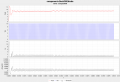there is an important difference in this model, the 2nd order differential $\frac{d^2V_{out}}{dt^2}$ term is deemed to be zero if computed diode current falls below zero (i.e. reverse biased). Without this assumption, it seemed the large oscillations would result.

the updated source with RKN solver is committed in the repository. no release is made
https://github.com/ag88/jbuckconv

Last edited:

#### ag-123

Joined Apr 28, 2017
273
more interesting results.
This is without including the diode in the calcs.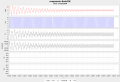Currently, models with the diode is incorrect. This is still the better one so far, by considering simply the switching Vin input.
Previous results imply a rising Vout, while a more accurate (RKN) solver shows an oscillating Vout.
However, the RKN solver is apparently less stable and tends to amplify accumulated errors.

#### ag-123

Joined Apr 28, 2017
273
after much work on the diode model, the diode model now more appropriately simulates a diode in the buck converter.
it is still unknown if it is after all correct

the BuckODEdiode2 simulation now looks like such, diode voltages and currents still looks odd.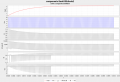The RKN solver produces this. I think the RKN solver possibly gives incorrect results. It tends to "amplify" signals when there is probably none, producing oscillations. I'm not too sure if this may be caused by cumulative errors. i.e. RKN solver is not stable and possibly produce incorrect results. But that it may be more 'accurate' in the sense that rather than showing a 'slow rise', it shows oscillations which may be a possibility when the converter is started up. These intervals are apparently short so I'd guess it is seldom observed in actual cases.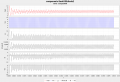#### ag-123

Joined Apr 28, 2017
273
https://github.com/ag88/jbuckconv
refactor: Vin into Vin,VinConf, add controller intf
- refactor Vin into Vin and VinConf interface
Vin becomes VinDefault class
- Vin has only a single method double getVin(double time)
used mainly by the ODE to get the current Vin
- VinConf interface is mainly for the gui panel to set
Vin level, frequency, duty cycle

- added Controller interface (controller.docontrol(Vin vin, BuckODE ode)
- ComputeCommMath and ComputeRKN calls controller interface
after each timestep iteration
This makes it easier to simulate a control scheme
currently no controller is implemented